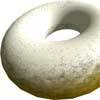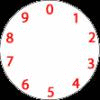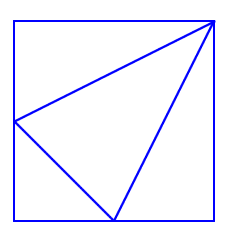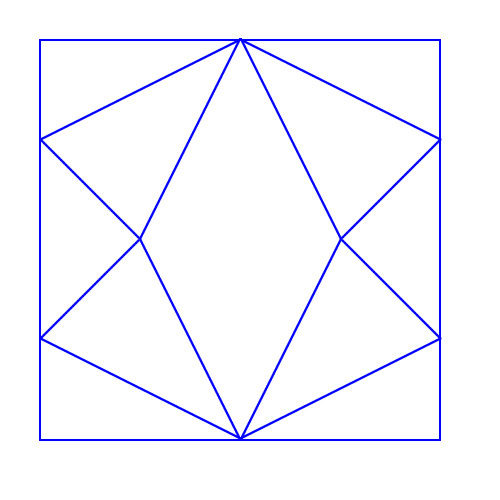#### You may also like### Doughnut

How can you cut a doughnut into 8 equal pieces with only three cuts of a knife?### Rectangle Tangle

The large rectangle is divided into a series of smaller quadrilaterals and triangles. Can you untangle what fractional part is represented by each of the shapes?### Dividing a Cake

Annie cut this numbered cake into 3 pieces with 3 cuts so that the numbers on each piece added to the same total. Where were the cuts and what fraction of the whole cake was each piece?

# Fraction Fascination

##### Age 7 to 11 Challenge Level:I drew this picture by drawing a line from the top right corner of a square to the midpoint of each of the opposite sides. Then I joined these two midpoints with another line.

Can you see four triangles in the square?

What fraction of the area of the square is each of these triangles?

Then I drew another picture:How is this made using the first square?

What is the shape that has been created in the middle of this larger square?

What fraction of the total area of the large square does this shape take up?